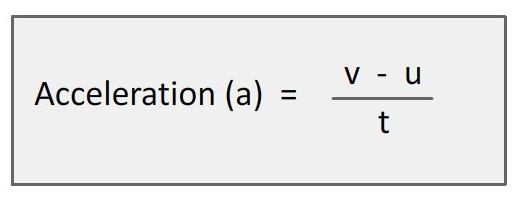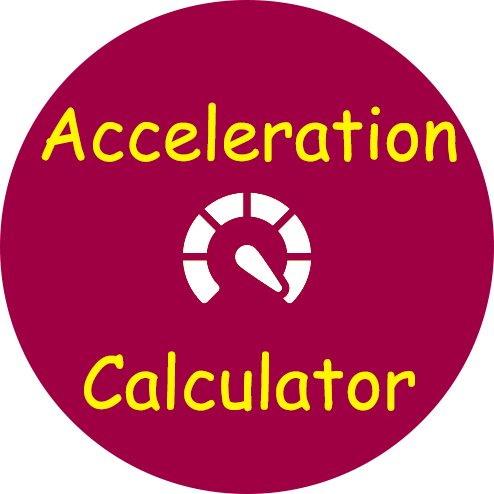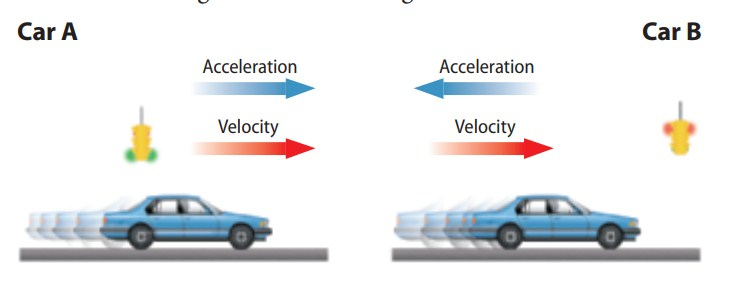# Acceleration Calculator

Use our acceleration calculator, a free online tool to calculate acceleration of object. Enter the initial velocity, final velocity and time pass during acceleration to find the object acceleration.### What is Acceleration of Object ?

Acceleration is the rate of change of velocity. When the velocity of an object changes, the object is accelerating. Remember that velocity is a measure that includes both speed and direction. Because of this, a change in velocity can be either a change in how fast something is moving or a change in the direction it is moving. Acceleration means that an object changes it speed, its direction, or both.

### How do you calculate acceleration?

Acceleration is the rate of change in velocity. To calculate acceleration, you first find the change in velocity. To find change in velocity subtract the beginning velocity of an object from the velocity at the end of its movement. Beginning velocity is calledthe initial velocity, or 'u'. Velocity at the end is called the final velocity, or 'v'

#### change in velocity = final velocity - initial velocity = v - u

If motion is in a straight line, the change in speed can be used to calculate the change in velocity. The change in speed is the final speed minus the initial speed.

To find acceleration, divide the change in velocity by the length of time during which the velocity changed.

acceleration (m/s2) = change in velocity (m/s) / time (s)

a = (v - u) / t

The SI unit for velocity is meters per second (m/s). To find acceleration, velocity is divided by the time in seconds (s). So, the unit for acceleration is m/s2.

#### Poistive and Negative Acceleration

The acceleration of an object that is speeding up different from that of an object that is slowing down. The acceleration of an object that is speeding up is always positive. The acceleration of an object that is slowing down is always negative.Acceleration has direction, just like velocity. In the figure above, both cars are accelerating because their speeds are changing. When a car’s acceleration and velocity are in the same direction, the speed increases and the acceleration is positive. Car A has positive acceleration. When a car is slowing down, the acceleration and velocity are in opposite directions. The acceleration is negative. Car B has negative acceleration.

### Spreading Knowledge Across the World

USA - United States of America  Canada  United Kingdom  Australia  New Zealand  South America  Brazil  Portugal  Netherland  South Africa  Ethiopia  Zambia  Singapore  Malaysia  India  China  UAE - Saudi Arabia  Qatar  Oman  Kuwait  Bahrain  Dubai  Israil  England  Scotland  Norway  Ireland  Denmark  France  Spain  Poland  and  many more....